| Next || Previous || Top |

## Example 10--Aragonite-Strontianite Solid Solution

PHREEQC has the capability to model multicomponent ideal and binary nonideal solid solutions. For ideal solid solutions, the activity of each end member solid is equal to its mole fraction. For nonideal solid solutions, the activity of each end member is the product of the mole fraction and an activity coefficient, which is determined from the mole fraction and Guggenheim excess free-energy parameters. Example 10 considers an aragonite (CaCO 3 )-strontianite (SrCO 3 ) solid solution and demonstrates how the composition of the solid solution and the aqueous phase change as strontium carbonate is added to an initially pure calcium carbonate system.

The example is derived from a diagram presented in Glynn and Parkhurst (1992). The equilibrium constants at 25 °C,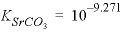and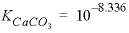, and the Guggenheim parameters,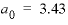and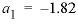, are derived from Plummer and Busenberg (1987). The input file is shown in table 29. The PHASES data block defines the log K s for aragonite and strontianite and overrides any data for these minerals that might be present in the database file. The SOLID_SOLUTIONS data block defines the unitless Guggenheim excess free-energy parameters and the initial composition of the solid solution, which is zero moles of aragonite and strontianite. Initial solution 1 is defined to be a calcium bicarbonate solution. The solution is then equilibrated with aragonite at nearly 1 atm partial pressure of carbon dioxide and saved as the new composition of solution 1.

 `TITLE Example 10.--Solid solution of strontianite and aragonite.` `PHASES` ` Strontianite` ` SrCO3 = CO3-2 + Sr+2` ` log_k -9.271` ` Aragonite` ` CaCO3 = CO3-2 + Ca+2` ` log_k -8.336` `END` `SOLID_SOLUTIONS 1` ` Ca(x)Sr(1-x)CO3 ` ` -comp1 Aragonite 0 ` ` -comp2 Strontianite 0 ` ` -Gugg_nondim 3.43 -1.82` `END` `SOLUTION 1` ` -units mmol/kgw` ` pH 5.93 charge` ` Ca 3.932` ` C 7.864` `EQUILIBRIUM_PHASES 1` ` CO2(g) -0.01265 10` ` Aragonite` `SAVE solution 1` `END` `#` `# Total of 0.00001 to 0.005 moles of SrCO3 added` `#` `USE solution 1` `USE solid_solution 1` `REACTION 1` ` SrCO3 1.0` ` .005 in 500 steps ` `PRINT` ` -reset false` ` -echo true` ` -user_print true` `USER_PRINT` `-start` ` 10 sum = (S_S("Strontianite") + S_S("Aragonite"))` ` 20 if sum = 0 THEN GOTO 110` ` 30 xb = S_S("Strontianite")/sum` ` 40 xc = S_S("Aragonite")/sum` ` 50 PRINT "Simulation number: ", SIM_NO` ` 60 PRINT "Reaction step number: ", STEP_NO` ` 70 PRINT "SrCO3 added: ", RXN` ` 80 PRINT "Log Sigma pi: ", LOG10 (ACT("CO3-2") * (ACT("Ca+2") + ACT("Sr+2")))` ` 90 PRINT "XAragonite: ", xc` ` 100 PRINT "XStrontianite: ", xb` ` 110 PRINT "XCa: ", TOT("Ca")/(TOT("Ca") + TOT("Sr"))` ` 120 PRINT "XSr: ", TOT("Sr")/(TOT("Ca") + TOT("Sr"))` ` 130 PRINT "Misc 1: ", MISC1("Ca(x)Sr(1-x)CO3")` ` 140 PRINT "Misc 2: ", MISC2("Ca(x)Sr(1-x)CO3")` `-end` `SELECTED_OUTPUT` ` -file ex10.sel` ` -reset false` ` -reaction true` `USER_PUNCH` `-head lg_SigmaPi X_Arag X_Stront X_Ca_aq X_Sr_aq mol_Misc1 mol_Misc2 \` ` mol_Arag mol_Stront` `-start` ` 10 sum = (S_S("Strontianite") + S_S("Aragonite"))` ` 20 if sum = 0 THEN GOTO 60` ` 30 xb = S_S("Strontianite")/(S_S("Strontianite") + S_S("Aragonite"))` ` 40 xc = S_S("Aragonite")/(S_S("Strontianite") + S_S("Aragonite"))` ` 50 REM Sigma Pi` ` 60 PUNCH LOG10(ACT("CO3-2") * (ACT("Ca+2") + ACT("Sr+2")))` ` 70 PUNCH xc # Mole fraction aragonite` ` 80 PUNCH xb # Mole fraction strontianite` ` 90 PUNCH TOT("Ca")/(TOT("Ca") + TOT("Sr")) # Mole aqueous calcium` ` 100 PUNCH TOT("Sr")/(TOT("Ca") + TOT("Sr")) # Mole aqueous strontium` ` 110 x1 = MISC1("Ca(x)Sr(1-x)CO3")` ` 120 x2 = MISC2("Ca(x)Sr(1-x)CO3")` ` 130 if (xb < x1 OR xb > x2) THEN GOTO 250` ` 140 nc = S_S("Aragonite")` ` 150 nb = S_S("Strontianite")` ` 160 mol2 = ((x1 - 1)/x1)*nb + nc` ` 170 mol2 = mol2 / ( ((x1 -1)/x1)*x2 + (1 - x2))` ` 180 mol1 = (nb - mol2*x2)/x1` ` 190 REM # Moles of misc. end members if in gap` ` 200 PUNCH mol1` ` 210 PUNCH mol2` ` 220 GOTO 300` ` 250 REM # Moles of misc. end members if not in gap` ` 260 PUNCH 1e-10` ` 270 PUNCH 1e-10` ` 300 PUNCH S_S("Aragonite") # Moles aragonite` ` 310 PUNCH S_S("Strontianite") # Moles Strontianite` `-end` `USER_GRAPH Example 10` ` -headings x_Aragonite x_Srontianite` ` -chart_title "Aragonite-Strontianite Solid Solution"` ` -axis_titles "Log(SrCO3 added, in moles)" "Log(Mole fraction of component)"` ` -axis_scale x_axis -5 1 1 1` ` -axis_scale y_axis -5 0.1 1 1` ` -connect_simulations true` ` -start` ` 10 sum = (S_S("Strontianite") + S_S("Aragonite"))` ` 20 IF sum = 0 THEN GOTO 70` ` 30 xb = S_S("Strontianite")/ sum` ` 40 xc = S_S("Aragonite")/ sum` ` 50 PLOT_XY LOG10(RXN), LOG10(xc), line_w = 2, symbol_size = 0` ` 60 PLOT_XY LOG10(RXN), LOG10(xb), line_w = 2, symbol_size = 0` ` 70 rem` ` -end` `END ` `#` `# Total of 0.005 to 0.1 moles of SrCO3 added` `#` `USE solution 1` `USE solid_solution 1` `REACTION 1` ` SrCO3 1.0` ` .1 in 20 steps ` `END ` `#` `# Total of 0.1 to 10 moles of SrCO3 added` `#` `USE solution 1` `USE solid_solution 1` `REACTION 1` ` SrCO3 1.0` ` 10.0 in 100 steps ` `END `

In the next simulation, solution 1 is brought together with the solid solution (USE keywords) and 5 millimoles of strontium carbonate are added in 500 steps (REACTION data block). The PRINT keyword data block excludes all default printing to the output file and includes only the printing defined in the USER_PRINT data block. The USER_PRINT data block specifies that the following information about the solid solution be printed to the output file after each reaction step: the simulation number, reaction-step number, amount of strontium carbonate added,(log of the sum of the ion activity products), mole fractions of strontianite and aragonite, aqueous mole fractions of calcium and strontium, and the composition of the two solids that exist within the miscibility gap. The SELECTED_OUTPUT data block defines the selected-output file to be ex10.sel , cancels any default printing to the selected-output file ( -reset false), and requests that the amount of reaction added at each step (as defined in the REACTION data block) be written to the selected-output file ( -reaction true). The USER_PUNCH data block prints additional columns of information to the selected-output file, including all of the information needed to make figure 11. Two additional simulations add successively larger amounts of strontium carbonate to the system up to a total addition of 10 mol.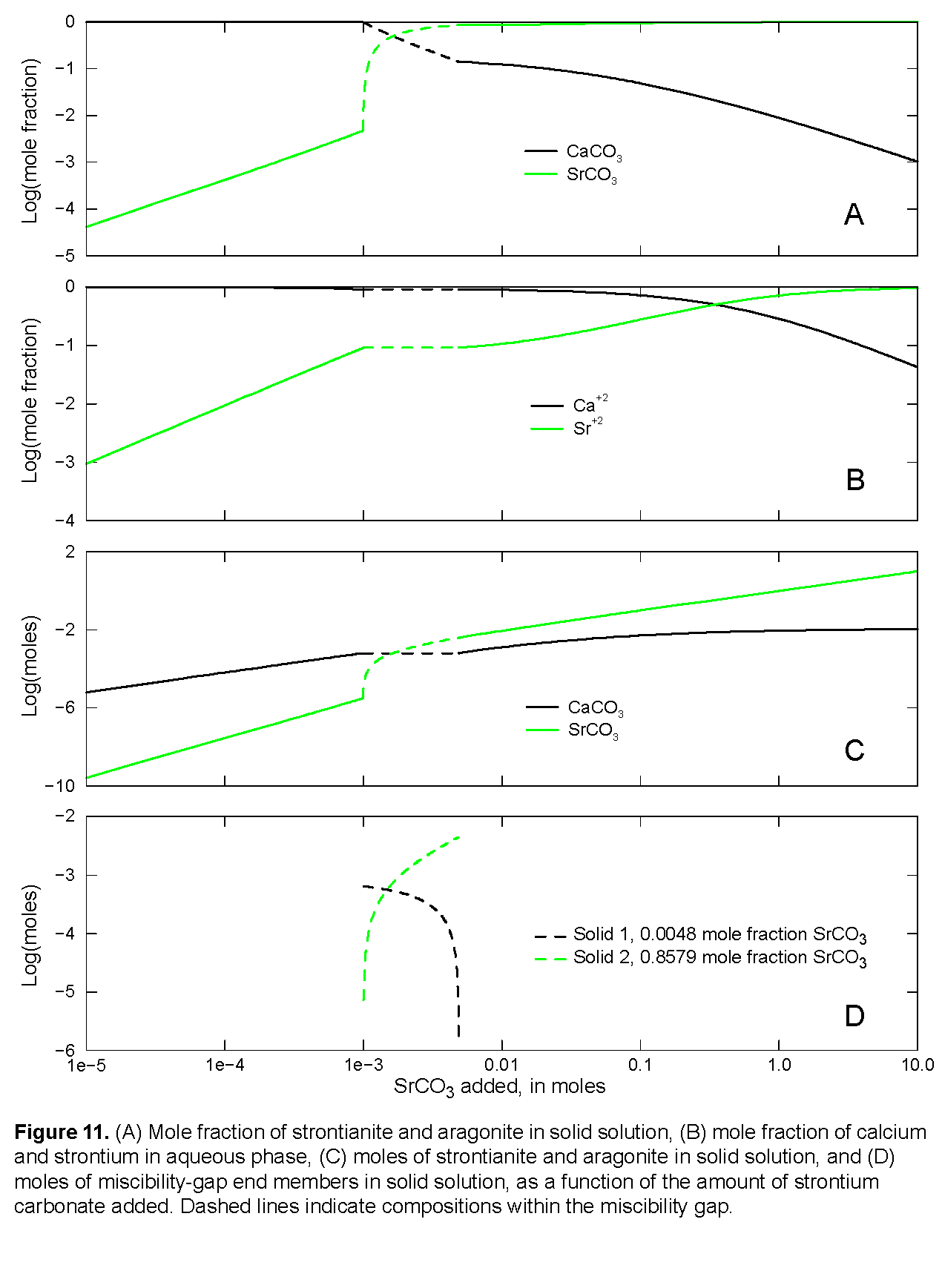The excess free-energy parameters describe a nonideal solid solution that has a miscibility gap. For compositions that fall within the miscibility gap, the activities of calcium and strontium within the aqueous phase remain fixed and are in equilibrium with solids of two compositions, one solid with a strontium mole fraction of 0.0048 and one solid with a strontium mole fraction of 0.8579. For the simulations of example 10, each incremental addition of strontium carbonate increases the mole fraction of strontium carbonate in the solid until about 0.001 mol of strontium carbonate have been added (fig. 11A). That point is the beginning of the miscibility gap (fig. 11) and the composition of the solid is 0.0048 strontium mole fraction. The next increments of strontium carbonate (up to 0.005 mol strontium carbonate added) produce constant mole fractions of calcium and strontium in the solution (fig. 11B) and equilibrium with both the miscibility-gap end members. However, the amounts of calcium carbonate and strontium carbonate in the solid phases (fig. 11C) and the amounts of each of the miscibility gap end members (fig. 11D) vary with the amount of strontium carbonate added. Finally, the end of the miscibility gap is reached after about 0.005 mol of strontium carbonate have been added. At this point, the solution is in equilibrium with a single solid with a strontium mole fraction of 0.8579. Addition of more strontium carbonate increases the mole fractions of strontium in the aqueous phase and in the solid solution until both mole fractions are nearly 1.0 after the addition of 10 mol of strontium carbonate.

| Next || Previous || Top |